## Coding-Decoding Tricks, Examples and Online Test

In this segment of common sense reasoning, secret messages or words have to be decoded. They are coded as per a definite pattern/ rule which should be identified 1st. Then the same is applied to decode another coded word. Under this segment you come across two types of coding letter coding and number coding. Based on these two types of coding-decoding various types of problems come your way. This chapter makes you familiar with every types of problems based on coding-decoding.

Example 1: If EODGH is the code for BLADE, what is the code for CRICKET?

Solution: FULFNHW

As,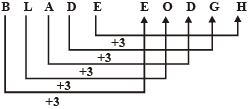Similarly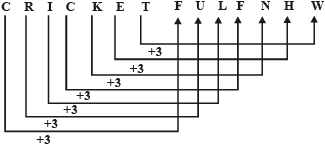Example 2: If EARTH is coded as 41590 and PALE as 2134, what is the code for PEARL?

Solution: 24153

codes for letters are: P=2, E=4, A=1, R=5 and L=3

Example 3: In a certain language, ‘put tir fin’ means ‘delicious juicy fruit’; ‘tie dip sig’ means ‘beautiful white lily’, and ‘sig lon fin’ means ‘lily and fruit’. What is the code for ‘and’?

Solution: ‘lon’

Common code from first and third statement for ‘fin’ is ‘fruit’. From second and third statement, ‘sig’ is ‘lily’. So ‘lon’ means ‘and’ in third statement.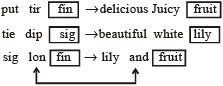### Type 1: Letter Coding

#### Methods of coding:

• (a) Skipping over the letter in the forward or backward direction.
• (b) Writing the letters of given word in reverse order in part or in whole.
• (c) Sometimes codes may be the position of those letters in alphabet.

Example 4: The word RUN is coded as SVO. What should be the code letters of LAY?

Solution: Here,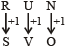Hence,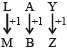∴ LAY should be MBZ.

Example 5: In a certain code DELHI is written as CDKGH. How is SUSPECT written in code?

Solution: Clearly, we can see that each letter of the word DELHI is moved one step backward to obtain the code.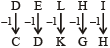Similarly, SUSPECT will be coded as RTRODBS.

Example 6: In a certain code COURAGE is written as UOCREGA. How will JOURNAL be written in the code.

Solution: Clearly, when COURAGE is coded, some letters are interchange with respect to their positions, i.e., odd position are interchanged.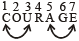Position of 1 changes to 3 and 3 to 1. Position of 5 changes to 7 and 7 to 5.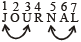can be coded as UOJRLAN.

### Type 2: Number Coding

This type of coding involves two types of cases :

I. When numerical code values are assigned to words:

Example 7 : If D = 4 and COVER = 63, then BASIS = ?

Solution: Clearly, in the given code, A = 1, B = 2, C = 3, ….. so that

COVER = 3 + 15 + 22 + 5 + 18 = 63

∴ BASIS = 2 + 1 + 19 + 9 + 19 = 50.

II. When alphabetical code values are assigned to the numbers.

Example 8: In a given code SISTER is coded as 535301. UNCLE as 84670 and BOY as 129. How is RUSTIC written in that code?

1. 633185
2. 185336
3. 363815
4. 581363

Solution: In this code the alphabets are coded as follows

 S I S T E R U N C L E B O Y 5 3 5 3 0 1 8 4 6 7 0 1 2 9

If we apply this method, the code comes out to be 185336

Example 9: In a certain code, 15789 is written as XTZAL and 2346 is written as NPSU. How is 23549 written in the code?

Solution: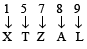and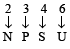So,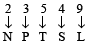∴  23549 can be written in code as NPTSL.

### Type 3: Substitution Coding

In substitution coding some particular objects are assigned as code names and then question is asked that is to be answered in the code language.

Example 10: If wall is called window, window is called door, door is called floor, floor is called roof and roof is called ventilator, what will a person stand on?

1. Door
2. Ventilator
3. Roof
4. Floor

Solution: Since a person stands on the floor and in the given code language floor is called roof.

Hence, roof will be correct answer.

### Type 4: Mixed letter coding

In this type of questions, three or more sentences are given in the coded language and the code for a particular word is asked. To find the code for the particular word, pickup two sentences bearing a common word and the common code word will mean that word. Proceeding in similar manner, we can be determined a particular code word for each word of given sentences.

Example 11: In a certain code language, ‘po ki top ma’ means ‘Usha is playing cards’. ‘kop ja ki ma’ means’ Asha is playing tennis’, ‘ki top sop ho’ means ‘they are playing football’ and ‘po sur kop’ means ‘cards and tennis’. Which word in that language means ‘Asha’?

1. ja
2. ma
3. kop
4. top

Solution. (a): Comparing relation in each of the statement, we get Asha is coded as ‘ja’.

### Type 5: Coding by Combinations of Letters, Numbers or Symbols

Directions: In each questions below is given a group of digits followed by four combinations of letters or symbols numbered 1, 2, 3 and 4. You have to find out which of the combinations correctly represents the group of digits based on the coding system and the conditions given below and mark the number of that combination as your answer. If none of the combinations correctly represents the group of digits, mark 5, i.e., ‘None of these’ as your answer.

 Digit 5 1 3 4 9 6 8 2 7 Symbol P A K % R @ d © M

Conditions:

• (i) If the first digit is odd and the last digit is even, the codes for the first and last digits are to be interchanged.
• (ii) If both the first and the last digits are even, both are to be coded as ♦
• (iii) If both the first and the last digits are odd, both are-to be coded as \$.

Example 12: What is the code of 215349?

2. ♦ APK%\$
3. \$APK%\$
5. None of these

Solution. (e):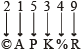Example 13: What is the code of 671254?

5. None of these

Solution. (c):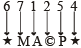Condition (ii) is applied

Example 14: What is the code of 813469?

1. DRK%R@
2. DAK%@ R
3. DAK%R@
5. None of these

Solution. (b):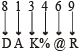Example 15: What is the code of 794821?

5. None of these

Solution. (d):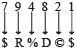Condition (iii) is applied

Example 16: What is the code of 591426?

5. None of these

Solution. (a):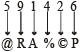Condition (i) is applied

### Type 6: Coded Letter of Word

Directions: These questions are based on code language which utilizes letters in the English alphabet. In each question, there is a word written in capital letter, with one letter underlined. For each letter in that word there is a code written in small letters. That code is denoted by 1, 2, 3, 4, and 5 not in the same order. You have to find out the exact code for the underlined letter in the word. The number of that code is the answer. Please note that the same letter appearing in other word(s) may be coded differently.

Example 17: QUITE

1. hj
2. su
3. tv
4. pr
5. df

Solution. (2): Each single letter is expressed as two letters, one behind and the other ahead of the given letter. Therefore, A becomes zb, B comes ac and so on.

Example 18: PRISM

1. R
2. O
3. H
4. Q
5. I

Solution. (4): All the letters of the word are coded as one letter behind.

Example 19: BEAST

1. c
2. w
3. d
4. h
5. v

Solution. (b): All the letters of the word are coded as three letters ahead.

Now, you must have been aware of the various kind of coding-decoding patterns. Point to be noted that the patterns discussed under this chapter are commonly known pattern/ basic patterns. So, if you practice hard, you find that after some times you become competent enough to solve coding-decoding problems even if certain changes are made in such problems to surprise or puzzle you.